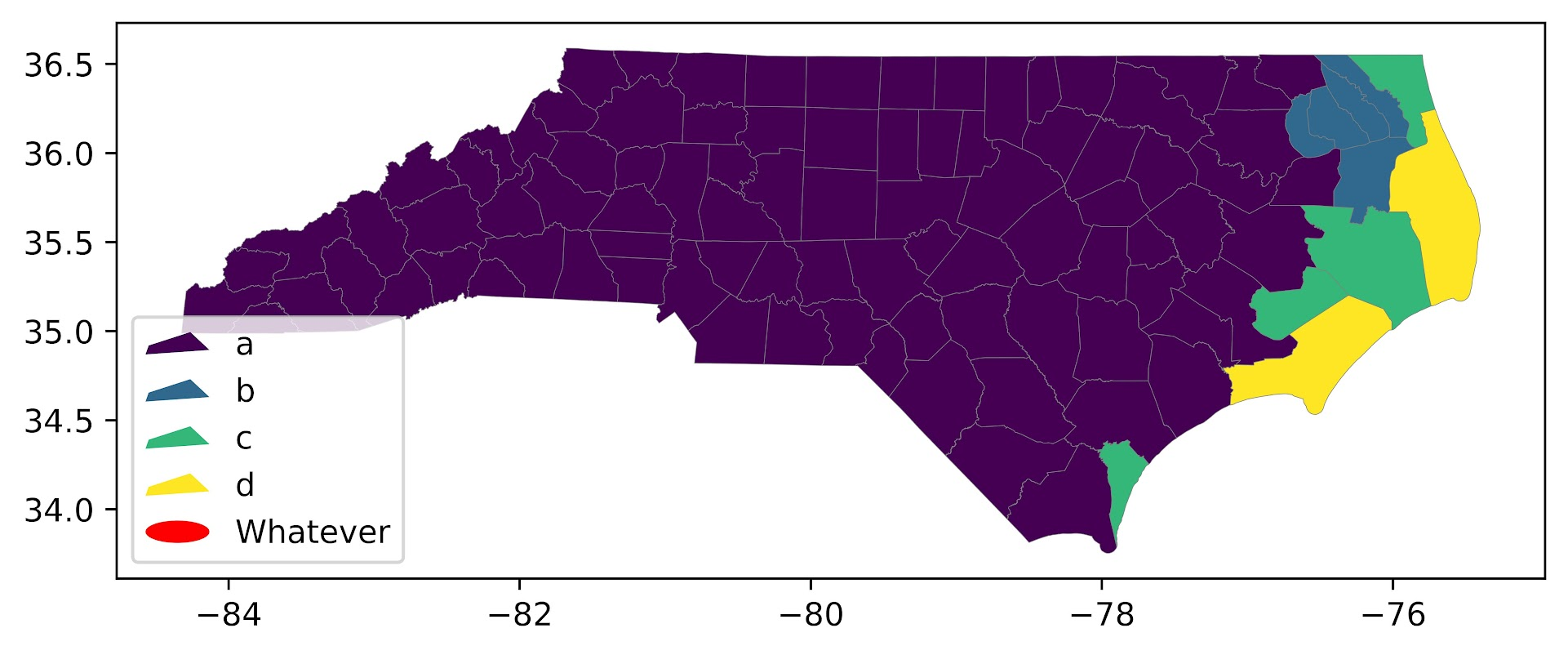# Legends in python

Legends in python and matplotlib can be a little tricky (it is quite a web of different objects). Here are a few notes I have collected over different projects. First, one thing python does not do, even if you name things the same, it does not combine them in a legend. First example will show superimposing lines and error bars – even though I only have two labels, they each get their own slot in the resulting legend.

``````import numpy as np
import geopandas as gpd

# Need a bunch of junk from matplotlib
import matplotlib
from matplotlib import pyplot as plt
from matplotlib import patches
from matplotlib.legend_handler import HandlerPatch

# Making simple fake data
x = [0,1,2,3]
y1 = np.array([1,1,1,1])
y1l = y1 - 0.5
y1h = y1 + 0.5

y2 = np.array([2,2,2,2])
y2l = y2 - 0.1
y2h = y2 + 0.1

# Default, does not combine legends
fig, ax = plt.subplots()
# First Line
ax.plot(x,y1,zorder=3, color='blue',alpha=0.9,label='y1')
ax.fill_between(x,y1l,y1h,alpha=0.2,zorder=2,color='blue',label='y1')
# Second Line
ax.plot(x,y2,zorder=3, color='k',alpha=0.9,label='y2')
ax.fill_between(x,y2l,y2h,alpha=0.2,zorder=2,color='k',label='y2')
ax.legend(bbox_to_anchor=(1.0, 0.5))
plt.savefig('Leg01.png', dpi=500, loc="center left", bbox_inches='tight')``````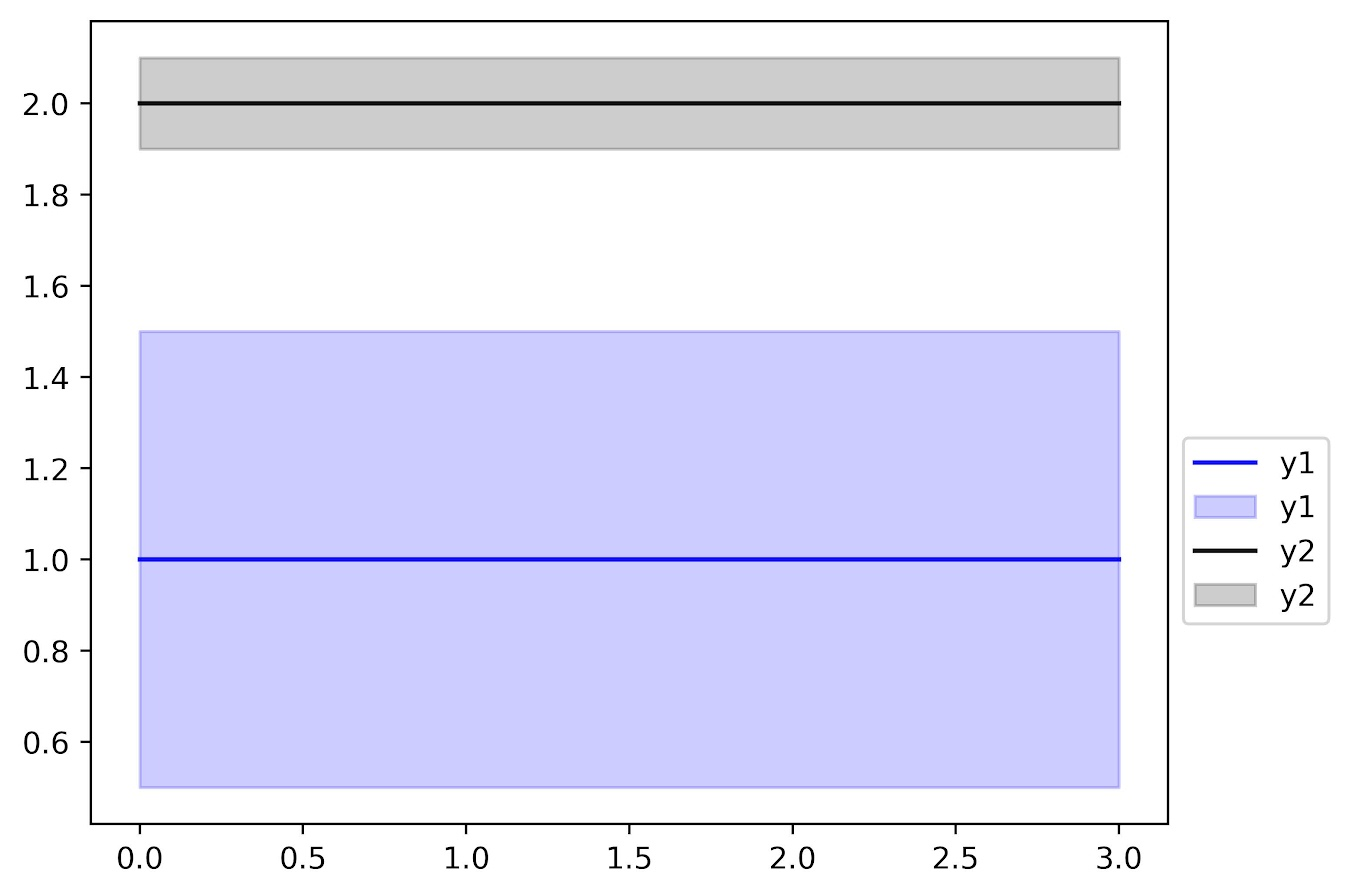You can combine the legend items by scooping out the original objects, here via the `ax.get_*` function to get the labels and the “handles”. You can think of handles as just points/lines/polygons that refer to individual parts of the legend. And so combining the lines and polygons together, we can make the legend how we want it.

``````fig, ax = plt.subplots()
# First Line
ax.plot(x,y1,zorder=3, color='blue',alpha=0.9,label='y1')
ax.fill_between(x,y1l,y1h,alpha=0.2,zorder=2,color='blue',label='y1')
# Second Line
ax.plot(x,y2,zorder=3, color='k',alpha=0.9,label='y2')
ax.fill_between(x,y2l,y2h,alpha=0.2,zorder=2,color='k',label='y2')
# Can combine legend items
handler, labeler = ax.get_legend_handles_labels()
hd = [(handler,handler),
(handler,handler)]
lab = ['y1','y2']
ax.legend(hd, lab, loc="center left", bbox_to_anchor=(1, 0.5))
plt.savefig('Leg02.png', dpi=500, bbox_inches='tight')``````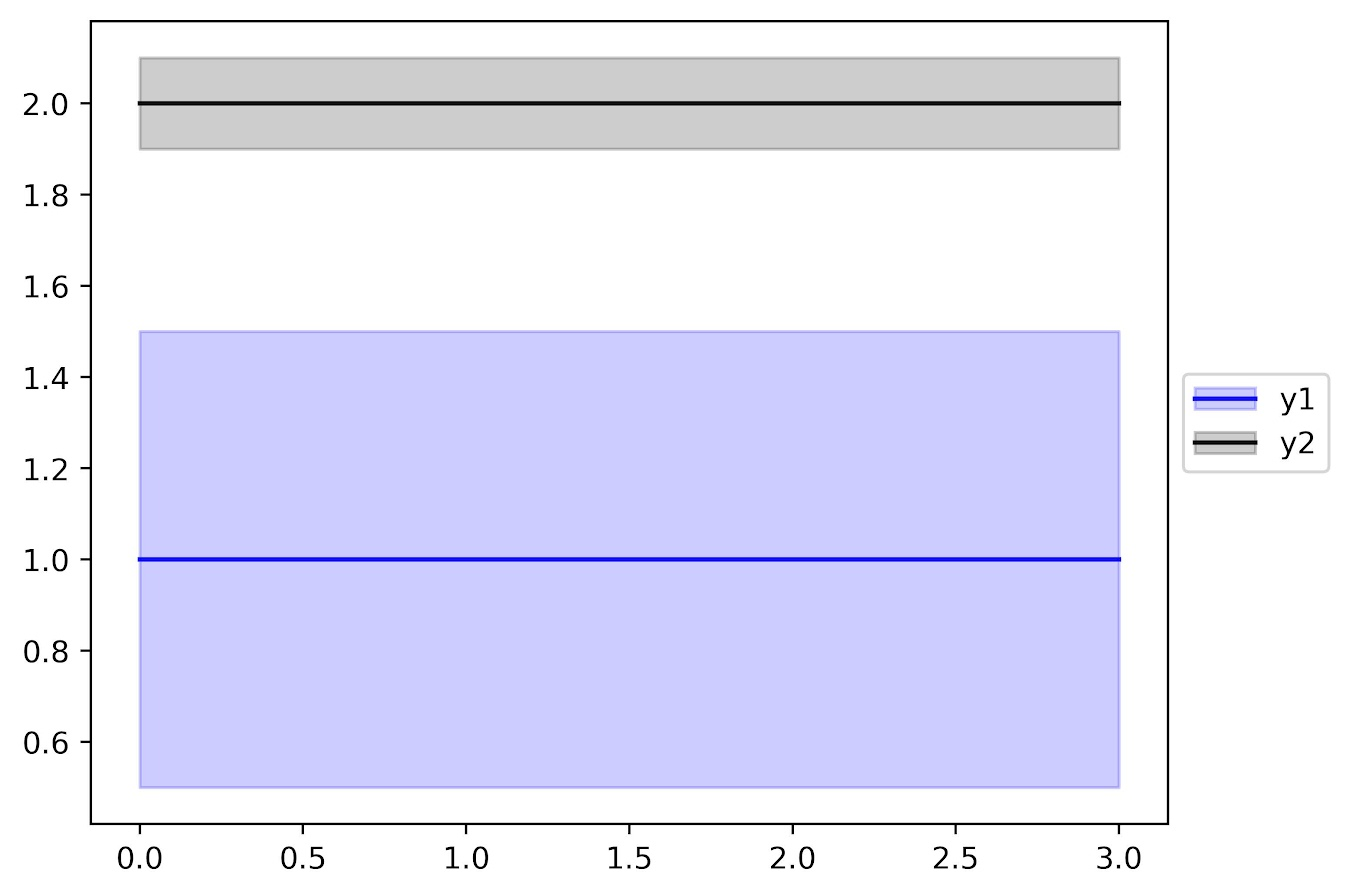I made a simple function `combo_legend(ax)` to combine items that have the same label in such matplotlib figures. So they do not need to per se be collections of similar items, just have the same text label.

``````# Can pass these to legend
def combo_legend(ax):
handler, labeler = ax.get_legend_handles_labels()
hd = []
labli = list(set(labeler))
for lab in labli:
comb = [h for h,l in zip(handler,labeler) if l == lab]
hd.append(tuple(comb))
return hd, labli

# Combo line/error/scatter
fig, ax = plt.subplots()
# First Line
ax.plot(x,y1,zorder=3, color='blue',alpha=0.9,label='y1')
ax.fill_between(x,y1l,y1h,alpha=0.2,zorder=2,color='blue',label='y1')
ax.scatter(x,y1,c='blue', edgecolor='white',
s=30,zorder=4,label='y1')
# Second Line
ax.plot(x,y2,zorder=3, color='k',alpha=0.9,label='y2')
ax.fill_between(x,y2l,y2h,alpha=0.2,zorder=2,color='k',label='y2')
hd, lab = combo_legend(ax)
ax.legend(hd, lab, loc="center left", bbox_to_anchor=(1, 0.5))
plt.savefig('Leg03.png', dpi=500, bbox_inches='tight')``````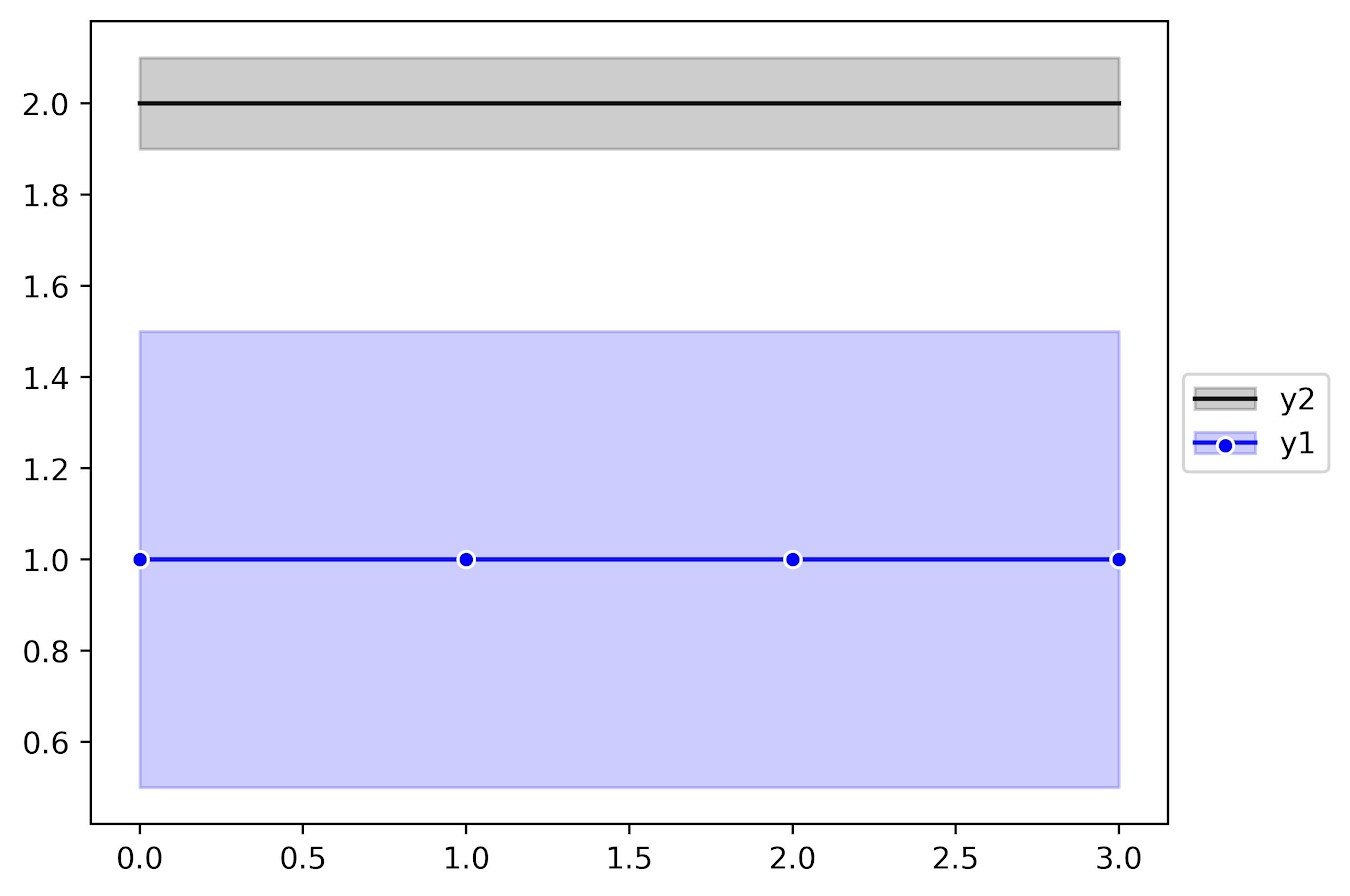Note that the `set()` call makes it so the order may not be how you want. You can just rearrange the objects you get back from `combo_legend` to sort them in the order you want.

Now for the second example in the post, is going to show some examples munging with geopandas objects/plots.

So default geopandas uses a continuous color ramp:

``````# Get counties for all US
county_url = r'https://www2.census.gov/geo/tiger/TIGER2019/COUNTY/tl_2019_us_county.zip'
# Get counties for North Carolina
nc = us_county[us_county['STATEFP'] == '37'].copy()
nc['wat_prop'] = nc['AWATER']/(nc['AWATER'] + nc['ALAND'])

# Plot proportion area water
fig, ax = plt.subplots(figsize=(8,4))
nc.plot(column='wat_prop', edgecolor='grey', linewidth=0.2, ax=ax, legend=True)
plt.savefig('Leg04.png', dpi=1000, bbox_inches='tight')``````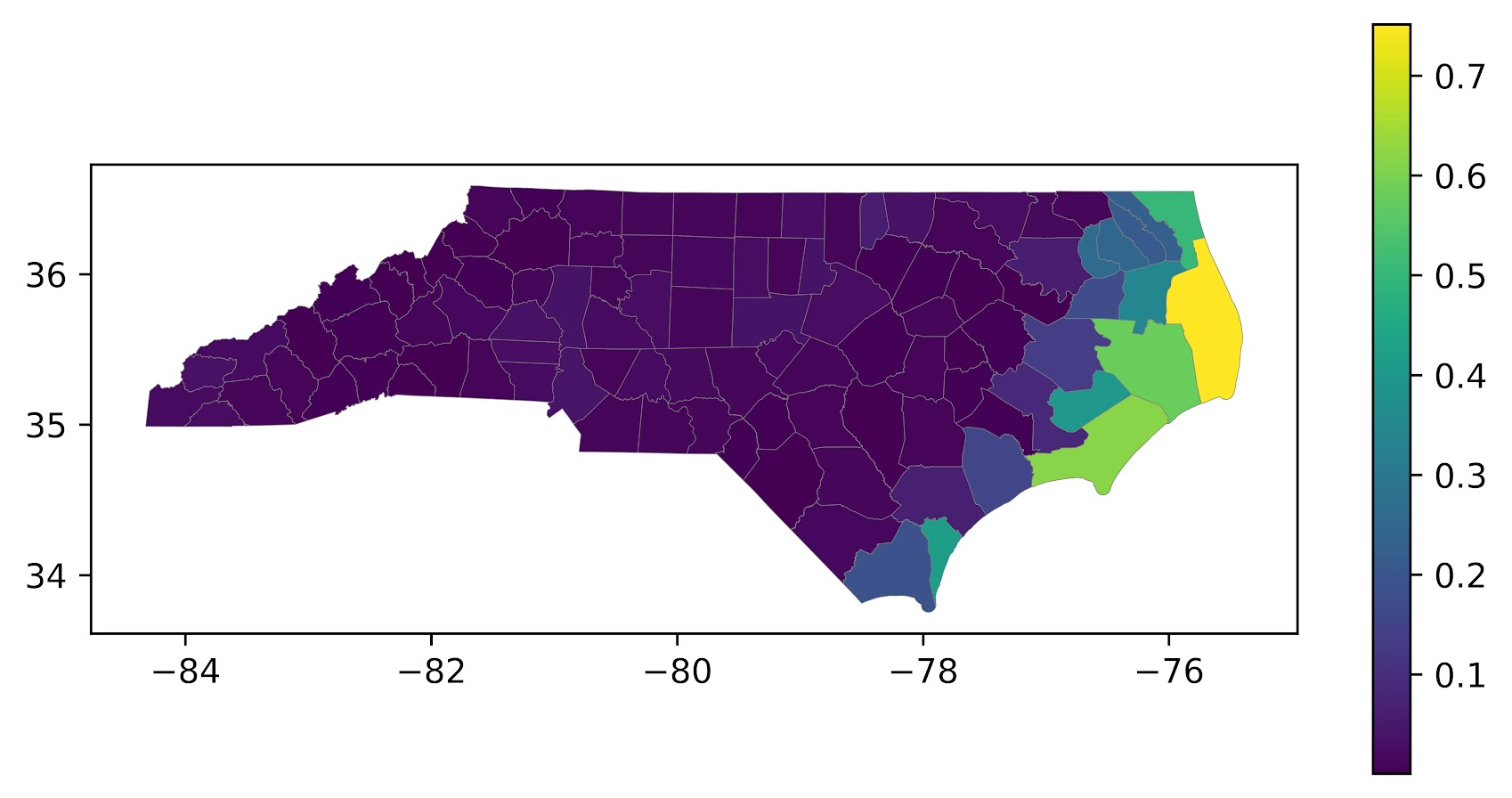I have a tough time with continuous color ramps. The geopandas mapping user guide has an example of making a nicer sized continuous legend, but overall I find making classed choropleth maps much easier in general. geopandas objects have a special set of arguments, where you can pass information to class the map and make a legend:

``````# Make the legend not the raster scale
bin_edge = np.arange(0.2,0.9,0.2)
leg_args = {'loc': 'lower left',
'prop': {'size': 9},
'title':'Prop. Water'}

fig, ax = plt.subplots(figsize=(8,4))
nc.plot(column='wat_prop',
scheme="User_Defined",
classification_kwds=dict(bins=bin_edge),
legend_kwds=leg_args,
edgecolor='grey',
linewidth=0.2,
ax=ax,
legend=True)
plt.savefig('Leg05.png', dpi=1000, bbox_inches='tight')``````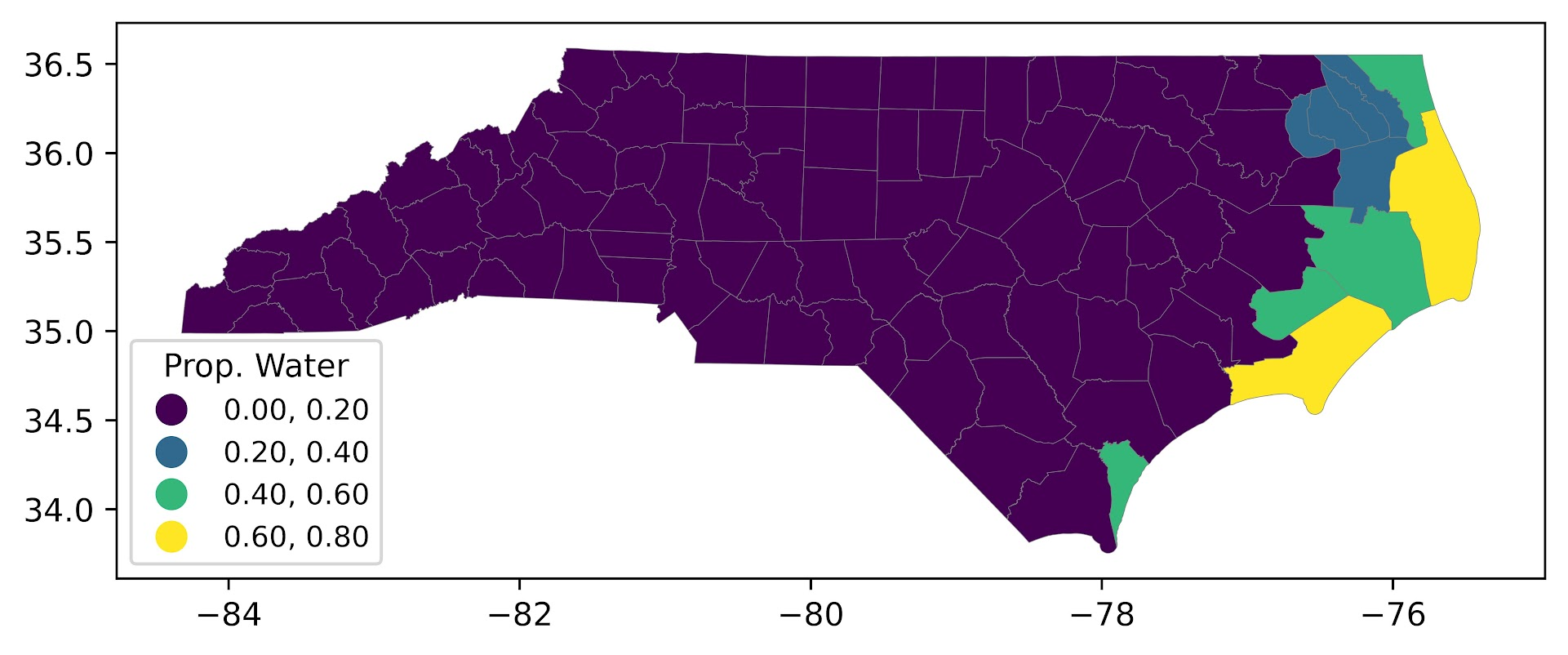But now we have circles. ArcGIS has the ability to use different glyphs in its legends, and it is common to use a blocky type glyph for geopolitical boundaries (which have unnatural straight lines).

If you go down the rabbit hole of geopandas objects, in this scenario the matplotlib handler is actually the same Line2D type object as if you call `ax.plot()`. So hacking together from a matplotlib doc tutorial, we can make a custom handler to draw our prespecified glyph. (Don’t take this as a nice example legend glyph, just threw something together very quick!) I also add in different labels to signify the boundary edges for the choropleth bins.

``````class MapHandler:
def legend_artist(self, legend, orig_handle, fontsize, handlebox):
x0, y0 = handlebox.xdescent, handlebox.ydescent
# Can handle lines or polygons
try:
face_col = orig_handle.get_markerfacecolor()
except:
face_col = orig_handle.get_facecolor()
width, height = handlebox.width, handlebox.height
# Ugly shape, can make your own!
xy = [[0,0],
[1,0.2],
[0.7,1],
[0.05,0.4]]
xy2 = []
for x,y in xy:
xt = x*width
yt = y*height
xy2.append([xt,yt])
patch = patches.Polygon(xy2, fc=face_col)
return patch

leg_args2 = leg_args.copy()
leg_args2['handler_map'] = {matplotlib.lines.Line2D: MapHandler()}
leg_args2['labels'] = ['[0.0 to 0.2)','[0.2 to 0.4)','[0.4 to 0.6)','[0.6 to 0.8]']

fig, ax = plt.subplots(figsize=(8,4))
nc.plot(column='wat_prop',
scheme="User_Defined",
classification_kwds=dict(bins=bin_edge),
legend_kwds=leg_args2,
edgecolor='grey',
linewidth=0.2,
ax=ax,
legend=True)
plt.savefig('Leg06.png', dpi=1000, bbox_inches='tight')``````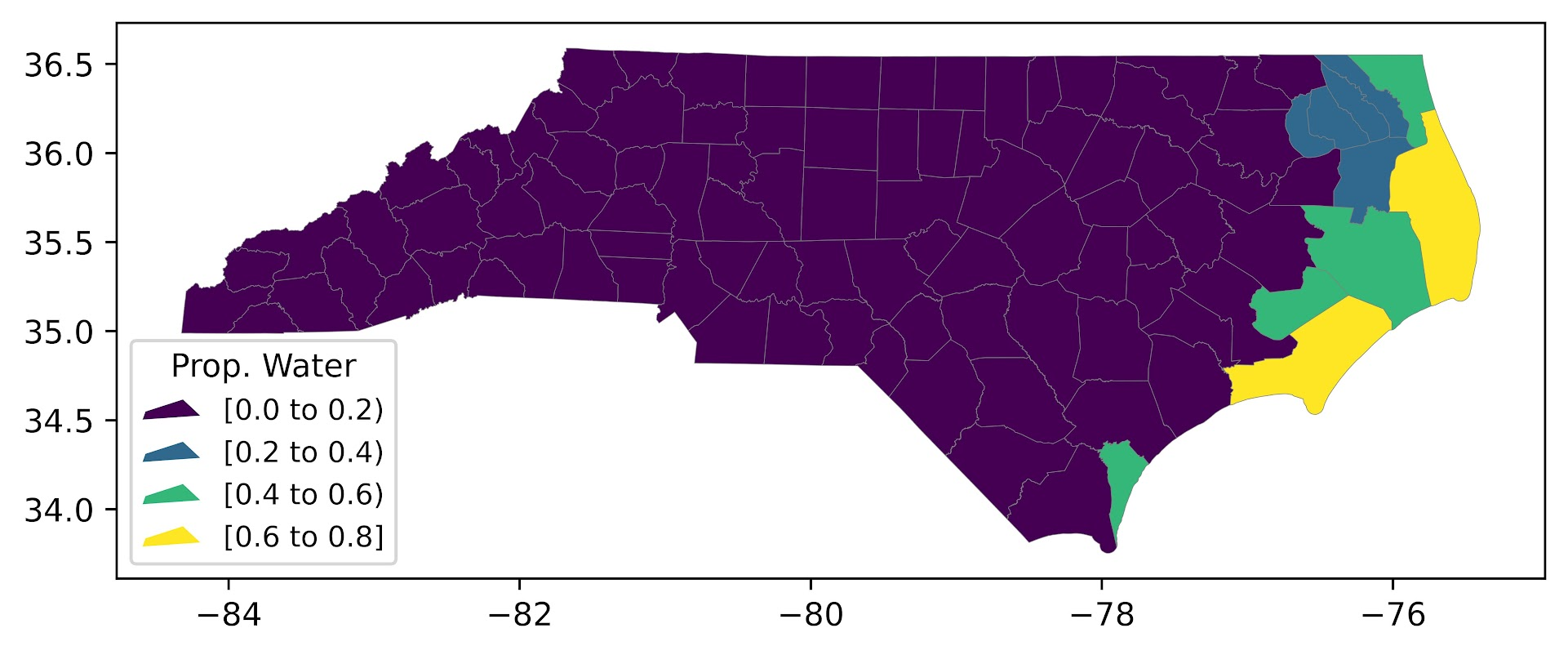This will be sufficient for many peoples choropleth map needs, but often times in maps you superimpose additional things, such as roads or other types of boundaries. So it may be necessary to go back into the legend and rebuild it entirely.

Here is an example of adding in something totally different, an ellipse, into the legend:

``````# Taken from that same matplotlib doc linked earlier
class HandlerEllipse(HandlerPatch):
def create_artists(self, legend, orig_handle,
xdescent, ydescent, width, height, fontsize, trans):
center = 0.5 * width - 0.5 * xdescent, 0.5 * height - 0.5 * ydescent
p = patches.Ellipse(xy=center, width=width + xdescent,
height=height + ydescent)
self.update_prop(p, orig_handle, legend)
p.set_transform(trans)
return [p]

fig, ax = plt.subplots(figsize=(8,4))
nc.plot(column='wat_prop',
scheme="User_Defined",
classification_kwds=dict(bins=bin_edge),
legend_kwds=leg_args2,
edgecolor='grey',
linewidth=0.2,
ax=ax,
legend=True)

# ax.get_legend_handles_labels() does not work here
leg = ax.get_legend()
handlers = leg.legendHandles
p = patches.Ellipse(xy=(0,0),width=2,height=1,facecolor='red')
handlers += [p]
new_labs = ['a','b','c','d','Whatever']
new_map = leg.get_legend_handler_map()
new_map[matplotlib.patches.Polygon] = MapHandler()
new_map[matplotlib.patches.Ellipse] = HandlerEllipse()
ax.legend(handlers, new_labs, handler_map=new_map, loc='lower left')
plt.savefig('Leg07.png', dpi=1000, bbox_inches='tight')``````